​JAVA从入门到精通-八大排序总结

2.1冒泡排序

· 俩俩交换，大的放在后面，第一次排序后最大值已在数组末尾。

· 因为俩俩交换，需要n-1趟排序，比如10个数，需要9趟排序

· 两个for循环，外层循环控制排序的趟数，内层循环控制比较的次数

o 每趟过后，比较的次数都应该要减1

· 优化：如果一趟排序后也没有交换位置，那么该数组已有序～

``` //外层循环是排序的趟数
for (int i = 0; i < arrays.length -1 ; i++) {
//每比较一趟就重新初始化为0
isChange = 0;

//内层循环是当前趟数需要比较的次数
for (int j = 0; j < arrays.length - i - 1; j++) {

//前一位与后一位与前一位比较，如果前一位比后一位要大，那么交换
if (arrays[j] > arrays[j + 1]) {
temp = arrays[j];
arrays[j] = arrays[j + 1];
arrays[j + 1] = temp;

//如果进到这里面了，说明发生置换了
isChange = 1;

}
}
//如果比较完一趟没有发生置换，那么说明已经排好序了，不需要再执行下去了
if (isChange == 0) {
break;
}

}
System.out.println("公众号Java3y" + arrays);```

2.2选择排序

· 找到数组中最大的元素，与数组最后一位元素交换

· 当只有一个数时，则不需要选择了，因此需要n-1趟排序，比如10个数，需要9趟排序

· 两个for循环，外层循环控制排序的趟数，内层循环找到当前趟数的最大值，随后与当前趟数组最后的一位元素交换

```    //外层循环控制需要排序的趟数
for (int i = 0; i < arrays.length - 1; i++) {

//新的趟数、将角标重新赋值为0
pos = 0;

//内层循环控制遍历数组的个数并得到最大数的角标
for (int j = 0; j < arrays.length - i; j++) {

if (arrays[j] > arrays[pos]) {
pos = j;
}

}
//交换
temp = arrays[pos];
arrays[pos] = arrays[arrays.length - 1 - i];
arrays[arrays.length - 1 - i] = temp;

}

System.out.println("公众号Java3y" + arrays);```

2.3插入排序

· 将一个元素插入到已有序的数组中，在初始时未知是否存在有序的数据，因此将元素第一个元素看成是有序的

· 与有序的数组进行比较，比它大则直接放入，比它小则移动数组元素的位置，找到个合适的位置插入

· 当只有一个数时，则不需要插入了，因此需要n-1趟排序，比如10个数，需要9趟排序

· 一个for循环内嵌一个while循环实现，外层for循环控制需要排序的趟数，while循环找到合适的插入位置(并且插入的位置不能小于0)

```  //临时变量
int temp;

//外层循环控制需要排序的趟数(从1开始因为将第0位看成了有序数据)
for (int i = 1; i < arrays.length; i++) {

temp = arrays[i];

//如果前一位(已排序的数据)比当前数据要大，那么就进入循环比较[参考第二趟排序]
while (i >= 1 && arrays[i - 1] > temp) {

//往后退一个位置，让当前数据与之前前位进行比较
arrays[i] = arrays[i - 1];

//不断往前，直到退出循环
i--;

}

//退出了循环说明找到了合适的位置了，将当前数据插入合适的位置中
arrays[i] = temp;

}
System.out.println("公众号Java3y" + arrays);```

2.4快速排序

· 在数组中找一个元素(节点)，比它小的放在节点的左边，比它大的放在节点右边。一趟下来，比节点小的在左边，比节点大的在右边。

· 不断执行这个操作....

· 快速排序用递归比较好写【如果不太熟悉递归的同学可到：递归就这么简单】。支点取中间，使用L和R表示数组的最小和最大位置

o 不断进行比较，直到找到比支点小(大)的数，随后交换，不断减小范围～

· 递归L到支点前一个元素(j)(执行相同的操作,同上)

· 递归支点后一个元素(i)到R元素(执行相同的操作,同上)

```/**
* 快速排序
*
* @param arr
* @param L   指向数组第一个元素
* @param R   指向数组最后一个元素
*/
public static void quickSort(int[] arr, int L, int R) {
int i = L;
int j = R;

//支点
int pivot = arr[(L + R) / 2];

//左右两端进行扫描，只要两端还没有交替，就一直扫描
while (i <= j) {

//寻找直到比支点大的数
while (pivot > arr[i])
i++;

//寻找直到比支点小的数
while (pivot < arr[j])
j--;

//此时已经分别找到了比支点小的数(右边)、比支点大的数(左边)，它们进行交换
if (i <= j) {
int temp = arr[i];
arr[i] = arr[j];
arr[j] = temp;
i++;
j--;
}
}
//上面一个while保证了第一趟排序支点的左边比支点小，支点的右边比支点大了。

//“左边”再做排序，直到左边剩下一个数(递归出口)
if (L < j)
quickSort(arr, L, j);

//“右边”再做排序，直到右边剩下一个数(递归出口)
if (i < R)
quickSort(arr, i, R);
}```

2.5归并排序

· 将两个已排好序的数组合并成一个有序的数组。

o 将元素分隔开来，看成是有序的数组，进行比较合并

o 不断拆分和合并，直到只有一个元素

· 在第一趟排序时实质是两个元素(看成是两个已有序的数组)来进行合并，不断执行这样的操作，最终数组有序

· 拆分左边，右边，合并...

```    public static void main(String[] args) {
int[] arrays = {9, 2, 5, 1, 3, 2, 9, 5, 2, 1, 8};
mergeSort(arrays, 0, arrays.length - 1);

System.out.println("公众号：Java3y" + arrays);

}

/**
* 归并排序
*
* @param arrays
* @param L      指向数组第一个元素
* @param R      指向数组最后一个元素
*/
public static void mergeSort(int[] arrays, int L, int R) {

//如果只有一个元素，那就不用排序了
if (L == R) {
return;
} else {

//取中间的数，进行拆分
int M = (L + R) / 2;

//左边的数不断进行拆分
mergeSort(arrays, L, M);

//右边的数不断进行拆分
mergeSort(arrays, M + 1, R);

//合并
merge(arrays, L, M + 1, R);

}
}

/**
* 合并数组
*
* @param arrays
* @param L      指向数组第一个元素
* @param M      指向数组分隔的元素
* @param R      指向数组最后的元素
*/
public static void merge(int[] arrays, int L, int M, int R) {

//左边的数组的大小
int[] leftArray = new int[M - L];

//右边的数组大小
int[] rightArray = new int[R - M + 1];

//往这两个数组填充数据
for (int i = L; i < M; i++) {
leftArray[i - L] = arrays[i];
}
for (int i = M; i <= R; i++) {
rightArray[i - M] = arrays[i];
}

int i = 0, j = 0;
// arrays数组的第一个元素
int  k = L;

//比较这两个数组的值，哪个小，就往数组上放
while (i < leftArray.length && j < rightArray.length) {

//谁比较小，谁将元素放入大数组中,移动指针，继续比较下一个
if (leftArray[i] < rightArray[j]) {
arrays[k] = leftArray[i];

i++;
k++;
} else {
arrays[k] = rightArray[j];
j++;
k++;
}
}

//如果左边的数组还没比较完，右边的数都已经完了，那么将左边的数抄到大数组中(剩下的都是大数字)
while (i < leftArray.length) {
arrays[k] = leftArray[i];

i++;
k++;
}
//如果右边的数组还没比较完，左边的数都已经完了，那么将右边的数抄到大数组中(剩下的都是大数字)
while (j < rightArray.length) {
arrays[k] = rightArray[j];

k++;
j++;
}
}```

2.6堆排序

· 堆排序使用到了完全二叉树的一个特性【不了解二叉树的同学可到：二叉树就这么简单学习一波】，根节点比左孩子和右孩子都要大，完成一次建堆的操作实质上是比较根节点和左孩子、右孩子的大小，大的交换到根节点上，直至最大的节点在树顶

· 随后与数组最后一位元素进行交换

· ......

· 只要左子树或右子树大于当前根节点，则替换。替换后会导致下面的子树发生了变化，因此同样需要进行比较，直至各个节点实现父>子这么一个条件

```public static void main(String[] args) {

int[] arrays = {6, 3, 8, 7, 5, 1, 2, 23, 4321, 432, 3,2,34234,2134,1234,5,132423, 234, 4, 2, 4, 1, 5, 2, 5};

for (int i = 0; i < arrays.length; i++) {

//每完成一次建堆就可以排除一个元素了
maxHeapify(arrays, arrays.length - i);

//交换
int temp = arrays;
arrays = arrays[(arrays.length - 1) - i];
arrays[(arrays.length - 1) - i] = temp;

}

System.out.println("公众号：Java3y" + arrays);

}

/**
* 完成一次建堆，最大值在堆的顶部(根节点)
*/
public static void maxHeapify(int[] arrays, int size) {

for (int i = size - 1; i >= 0; i--) {
heapify(arrays, i, size);
}

}

/**
* 建堆
*
* @param arrays          看作是完全二叉树
* @param currentRootNode 当前父节点位置
* @param size            节点总数
*/
public static void heapify(int[] arrays, int currentRootNode, int size) {

if (currentRootNode < size) {
//左子树和右字数的位置
int left = 2 * currentRootNode + 1;
int right = 2 * currentRootNode + 2;

//把当前父节点位置看成是最大的
int max = currentRootNode;

if (left < size) {
//如果比当前根元素要大，记录它的位置
if (arrays[max] < arrays[left]) {
max = left;
}
}
if (right < size) {
//如果比当前根元素要大，记录它的位置
if (arrays[max] < arrays[right]) {
max = right;
}
}
//如果最大的不是根元素位置，那么就交换
if (max != currentRootNode) {
int temp = arrays[max];
arrays[max] = arrays[currentRootNode];
arrays[currentRootNode] = temp;

//继续比较，直到完成一次建堆
heapify(arrays, max, size);
}
}
}```

2.7希尔排序

· 希尔排序实质上就是插入排序的增强版，希尔排序将数组分隔成n组来进行插入排序，直至该数组宏观上有序，最后再进行插入排序时就不用移动那么多次位置了～

· 希尔增量一般是gap = gap / 2，只是比普通版插入排序多了这么一个for循环罢了，难度并不大

``` /**
* 希尔排序
*
* @param arrays
*/
public static void shellSort(int[] arrays) {

//增量每次都/2
for (int step = arrays.length / 2; step > 0; step /= 2) {

//从增量那组开始进行插入排序，直至完毕
for (int i = step; i < arrays.length; i++) {

int j = i;
int temp = arrays[j];

// j - step 就是代表与它同组隔壁的元素
while (j - step >= 0 && arrays[j - step] > temp) {
arrays[j] = arrays[j - step];
j = j - step;
}
arrays[j] = temp;
}
}

}```

2.8基数排序

· 基数排序(桶排序)：将数字切割成个、十、百、千位放入到不同的桶子里，放一次就按桶子顺序回收一次，直至最大位数的数字放完～那么该数组就有序了

· 先找到数组的最大值，然后根据最大值/10来作为循环的条件(只要>0，那么就说明还有位数)

· 将个位、十位、...分配到桶子上，每分配一次就回收一次

```  public static void main(String[] args) {

int[] arrays = {6, 4322, 432, 344, 55, 234, 45, 243, 5, 2, 4, 5, 6, 7, 3245, 345, 345, 234, 68, 65};

System.out.println("公众号：Java3y" + arrays);

}

public static void radixSort(int[] arrays) {

int max = findMax(arrays, 0, arrays.length - 1);

//需要遍历的次数由数组最大值的位数来决定
for (int i = 1; max / i > 0; i = i * 10) {

int[][] buckets = new int[arrays.length];

//获取每一位数字(个、十、百、千位...分配到桶子里)
for (int j = 0; j < arrays.length; j++) {

int num = (arrays[j] / i) % 10;

//将其放入桶子里
buckets[j][num] = arrays[j];
}

//回收桶子里的元素
int k = 0;

//有10个桶子
for (int j = 0; j < 10; j++) {
//对每个桶子里的元素进行回收
for (int l = 0; l < arrays.length ; l++) {

//如果桶子里面有元素就回收(数据初始化会为0)
if (buckets[l][j] != 0) {
arrays[k++] = buckets[l][j];

}

}

}

}
}

/**
* 递归，找出数组最大的值
*
* @param arrays 数组
* @param L      左边界，第一个数
* @param R      右边界，数组的长度
* @return
*/

public static int findMax(int[] arrays, int L, int R) {

//如果该数组只有一个数，那么最大的就是该数组第一个值了
if (L == R) {
return arrays[L];
} else {

int a = arrays[L];
int b = findMax(arrays, L + 1, R);//找出整体的最大值

if (a > b) {
return a;
} else {
return b;
}
}
}```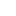喜欢 | 0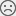不喜欢 | 0

• 11
文章
• 1522
人气
• 83%
受欢迎度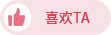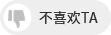•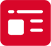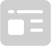索取资料
•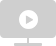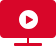答疑解惑
•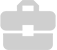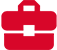技术交流
•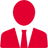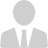职业测评
•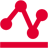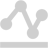面试技巧
•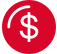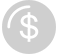高薪秘笈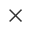Java全栈开发
WEB前端+H5沪公网安备 31011502005948号    ICP许可  沪B2-20190160